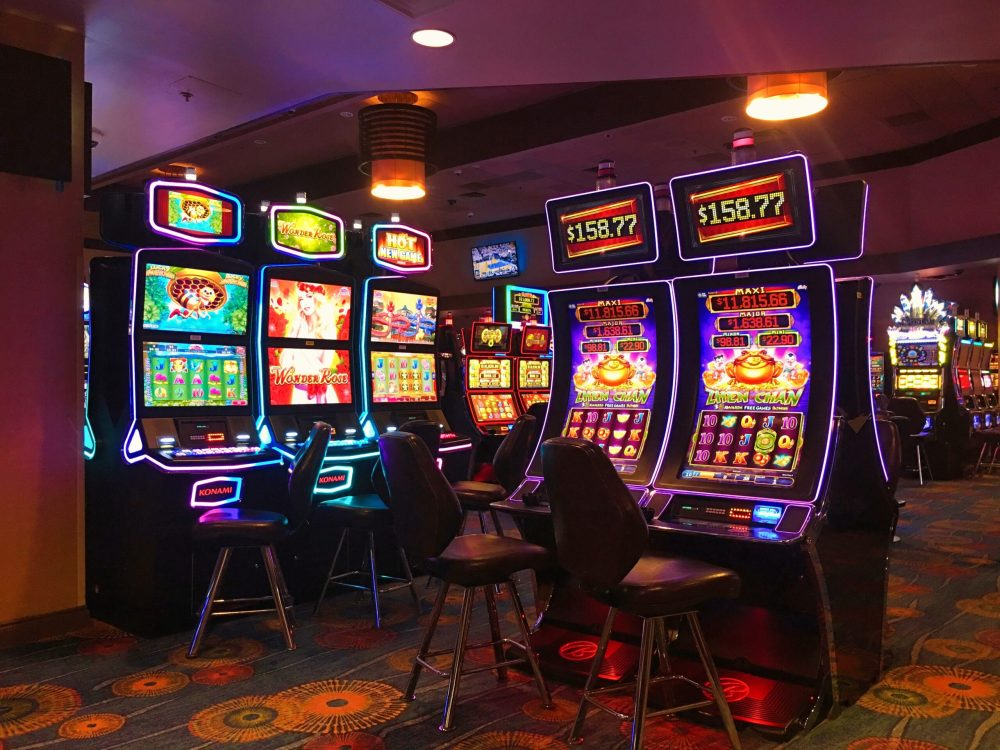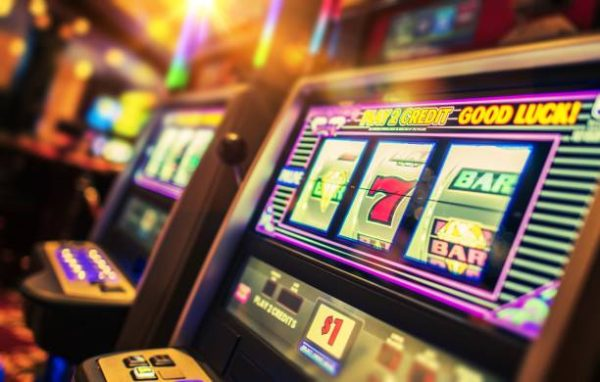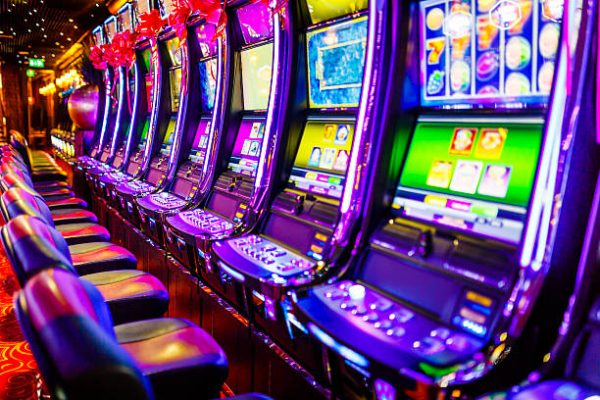December 11, 2023Slot machines are a popular attraction at casinos across the world. From the classic three-reel machines to the latest video slots, the flashing lights and dinging sounds of modern-day machines create a tempting allure for players. However, underneath the surface, these machines are designed with complex algorithms and probabilities that dictate the game’s outcome. In this article, we will delve into slot machine mathematics and explore the algorithms and probabilities behind these games.

The basics of slot machine mathematics

The foundation on which slot machine mathematics is built is the random number generator (RNG). This program generates millions of numbers every second, even when the machine is not being used. When a player spins the reels, the RNG stops on a number, which determines the outcome of the game. Whether a player wins or loses is determined by a combination of luck and probability.The impact of probability on slot machines

One of the most essential aspects of slot machine mathematics is probability theory. Probability theory is a branch of mathematics that helps to understand the likelihood of an event occurring. In the case of slot machines, it is used to determine the odds of a particular combination of symbols popping up on the reels. This probability is calculated using a mathematical formula, which takes into account the number of symbols on each reel, the number of reels, and the total number of possible combinations.

Slot machine algorithms

Apart from probability, slot machines also use complex algorithms that determine when the machines will pay out jackpots. Most modern slot machines are designed to pay out a certain percentage of the money wagered by players over the long term. This is known as the Return to Player (RTP) percentage. The RTP percentage can range from around 85% to 98%, with the higher percentage being more favorable to the player.

Slot machine algorithms could be further classified into two types- deterministic and stochastic. The deterministic algorithms work on the principle of exact knowledge, while stochastic algorithms use probability and statistics to determine the outcome of the game.The importance of RTP

As mentioned earlier, the RTP percentage is an essential aspect of slot machine mathematics. It determines how much money players can expect to win in the long run. For instance, a slot machine with an RTP percentage of 95% would pay back 95 cents for every dollar wagered over the long term. It is important to note that this percentage is calculated over millions of spins and not just a single session. Therefore, a player’s chances of winning in any single spin are not dependent on the machine’s RTP percentage.

Slot machines have come a long way since the first slot machine was invented in 1895. They have evolved from simple machines to complex electronic games with sophisticated algorithms and probabilities. Understanding the mathematics behind these machines is key to maximising your chances of winning in the long term. Whether you’re a seasoned player or a novice, knowing the inner workings of slot machines will make your gaming experience more enjoyable and rewarding.